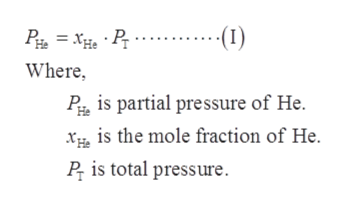# A mixture of He and CO2  is placed in a 7.5L flask at 22C. The partial pressure of the He is 2.2 atm and the partial pressure of the CO2 is 1.9 atm. What is the mole fraction of He?

Question
61 views

A mixture of He and CO2  is placed in a 7.5L flask at 22C. The partial pressure of the He is 2.2 atm and the partial pressure of the CO2 is 1.9 atm. What is the mole fraction of He?

check_circle

star
star
star
star
star
1 Rating
Step 1

Given data:

Step 2

The total pressure is the sum of partial pressure of He and CO2. The total pressure is calculated as shown below.

Step 3

The mole fraction of helium (He) is calcu...help_outlineImage Transcriptionclose(1) Where P is partial pressure of He xis the mole fraction of He P is total pressure fullscreen

### Want to see the full answer?

See Solution

#### Want to see this answer and more?

Solutions are written by subject experts who are available 24/7. Questions are typically answered within 1 hour.*

See Solution
*Response times may vary by subject and question.
Tagged in

### Physical Chemistry